Newton’s laws of motionPage 2

WATCH ALL SLIDES

Slide 11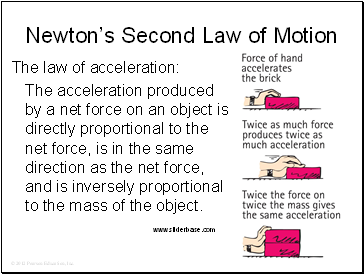Newton’s Second Law of Motion

The law of acceleration:

The acceleration produced by a net force on an object is directly proportional to the net force, is in the same direction as the net force, and is inversely proportional to the mass of the object.

Slide 12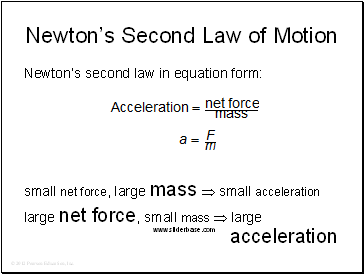Newton’s Second Law of Motion

Newton’s second law in equation form:

small net force, large mass  small acceleration

large net force, small mass  large acceleration

Slide 13Consider a cart pushed along a track with a certain force. If the force remains the same while the mass of the cart decreases to half, the acceleration of the cart

A. remains the same.

halves.

doubles.

changes unpredictably.

Newton’s Second Law of Motion

Slide 14Consider a cart pushed along a track with a certain force. If the force remains the same while the mass of the cart decreases to half, the acceleration of the cart

A. remains the same.

halves.

doubles.

changes unpredictably.

Newton’s Second Law of Motion

Slide 15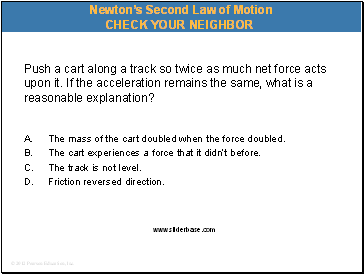Push a cart along a track so twice as much net force acts upon it. If the acceleration remains the same, what is a reasonable explanation?

A. The mass of the cart doubled when the force doubled.

The cart experiences a force that it didn’t before.

The track is not level.

Friction reversed direction.

Newton’s Second Law of Motion

Slide 16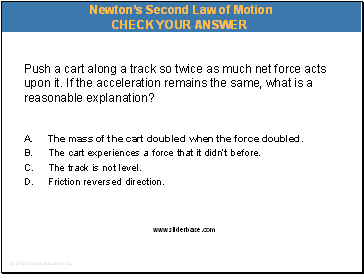Push a cart along a track so twice as much net force acts upon it. If the acceleration remains the same, what is a reasonable explanation?

A. The mass of the cart doubled when the force doubled.

The cart experiences a force that it didn’t before.

The track is not level.

Friction reversed direction.

Newton’s Second Law of Motion

Slide 17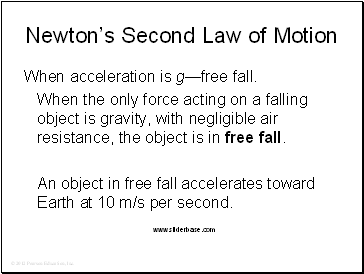Newton’s Second Law of Motion

When acceleration is g—free fall.

When the only force acting on a falling object is gravity, with negligible air resistance, the object is in free fall.

An object in free fall accelerates toward Earth at 10 m/s per second.

Slide 18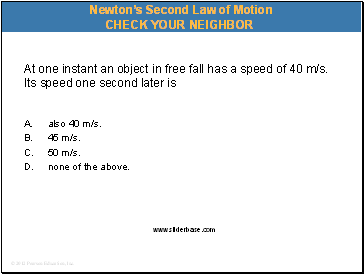At one instant an object in free fall has a speed of 40 m/s. Its speed one second later is

Go to page:
1  2  3  4  5  6  7  8  9